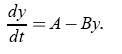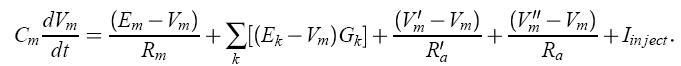## Help with implementing exponential euler in own simulator

### Help with implementing exponential euler in own simulator

Hi

I'm developing a simple simulation environment for compartmental models and want to use the exponential euler as numerical integration method.

My main problem is that: exponential Euler assumes an equation in the form ofhowever, I just can't work out, how the equationcould fit into that pattern.

All literature I found jump from the first equation directly to the second (or the other way around) but they don't explain of which values A and B consist of.

I hope someone can help me with that problem since I really want to understand what I'm doing.

Regards